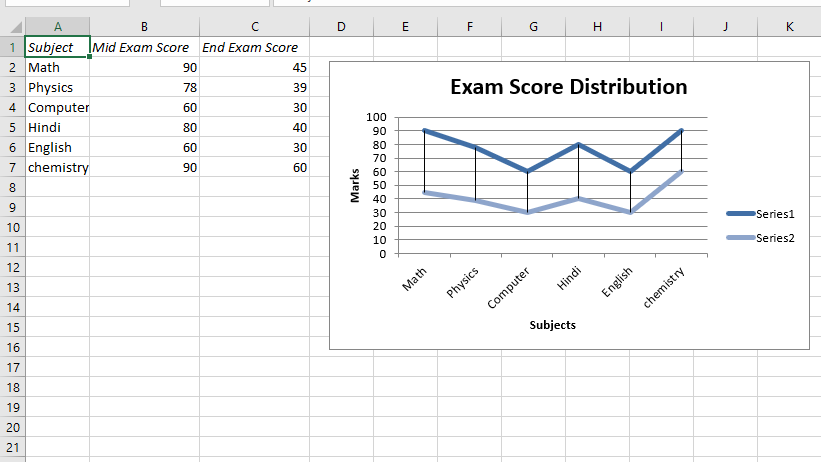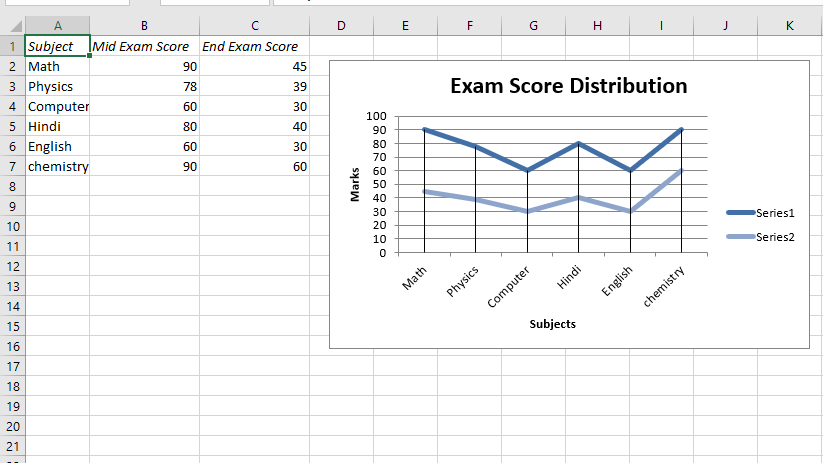# Python | Plotting charts in excel sheet with data tools using XlsxWriter module | Set – 2

Prerequisite: Create and Write on an excel sheet

`XlsxWriter` is a Python library using which one can perform multiple operations on excel files like creating, writing, arithmetic operations and plotting graphs. Let’s see how to plot charts with different types of Data Tools using realtime data.

Charts are composed of at least one series of one or more data points. Series themselves are comprised of references to cell ranges. For plotting the charts on an excel sheet, firstly, create chart object of specific chart type( i.e Line chart etc.). After creating chart objects, insert data in it and lastly, add that chart object in the sheet object.

Code #1 : Plot a Chart with Up-Down Bars.
For plotting this type of chart on an excel sheet, use `set_up_down_bars()` method of the chart object.

 `# import xlsxwriter module ` `import` `xlsxwriter ` ` `  `# Workbook() takes one, non-optional, argument    ` `# which is the filename that we want to create.  ` `workbook ``=` `xlsxwriter.Workbook(``'Example4_chart.xlsx'``) ` ` `  `# The workbook object is then used to add new    ` `# worksheet via the add_worksheet() method.  ` `worksheet ``=` `workbook.add_worksheet() ` ` `  `# Create a new Format object to formats cells  ` `# in worksheets using add_format() method .  ` `   `  `# here we create italic format object  ` `italic ``=` `workbook.add_format({``'italic'``: ``1``}) ` ` `  `# Add the worksheet data that the charts will refer to. ` `Data1 ``=` `[``'Subject'``, ``'Mid Exam Score'``, ``'End Exam Score'``] ` `Data2 ``=` `[ ` `    ``[``"Math"``, ``"Physics"``, ``"Computer"``,  ` `     ``"Hindi"``, ``"English"``, ``"chemistry"``], ` `    ``[``90``, ``78``, ``60``, ``80``, ``60``, ``90``], ` `    ``[``45``, ``39``, ``30``, ``40``, ``30``, ``60``] ` `] ` ` `  `# Write a row of data starting from 'A1'  ` `# with bold format .  ` `worksheet.write_row(``'A1'``, Data1, italic) ` ` `  `# Write a column of data starting from   ` `# 'A2', 'B2', 'C2' respectively  ` `worksheet.write_column(``'A2'``, Data2[``0``]) ` `worksheet.write_column(``'B2'``, Data2[``1``]) ` `worksheet.write_column(``'C2'``, Data2[``2``]) ` ` `  `# set the wdith of B and C column ` `worksheet.set_column(``'B:C'``, ``15``) ` ` `  `# Create a chart object that can be added  ` `# to a worksheet using add_chart() method.  ` ` `  `# here we create a line chart object . ` `chart1 ``=` `workbook.add_chart({``'type'``: ``'line'``}) ` ` `  `# Adding the Up-Down Bars. ` `chart1.set_up_down_bars() ` ` `  `# Add a data series to a chart   ` `# using add_series method.   ` `     `  `# Configure the first series.   ` `# = Sheet1 !\$A\$1 is equivalent to ['Sheet1', 0, 0].  ` `   `  `# note : spaces is not inserted in b / w  ` `# = and Sheet1, Sheet1 and !  ` `# if space is inserted it throws warning.  ` `chart1.add_series({  ` `    ``'categories'``: ``'= Sheet1 !\$A\$2:\$A\$7'``,  ` `    ``'values'``:     ``'= Sheet1 !\$B\$2:\$B\$7'``,  ` `})  ` `   `  `# Configure the second series. ` `chart1.add_series({  ` `    ``'categories'``: ``'= Sheet1 !\$A\$2:\$A\$7'``,  ` `    ``'values'``:     ``'= Sheet1 !\$C\$2:\$C\$7'``,  ` `})  ` `   `  `# Add a chart title.  ` `chart1.set_title({``'name'``: ``'Exam Score Distribution'``})  ` `   `  `# Add x-axis label   ` `chart1.set_x_axis({``'name'``: ``'Subjects'``})   ` `     `  `# Add y-axis label   ` `chart1.set_y_axis({``'name'``: ``'Marks'``})  ` `   `  `# Set an Excel chart style.   ` `chart1.set_style(``11``)  ` `   `  `# add chart to the worksheet with given  ` `# offset values at the top-left corner of  ` `# a chart is anchored to cell D2   ` `worksheet.insert_chart(``'D2'``, chart1,   ` `    ``{``'x_offset'``: ``20``, ``'y_offset'``: ``5``})  ` `   `  `# Finally, close the Excel file    ` `# via the close() method.    ` `workbook.close()  `

Output :Code #2 : Plot a Chart with High-Low Lines.
For plotting this type of chart on an excel sheet, use `set_high_low_lines()` method of the chart object.

 `# import xlsxwriter module ` `import` `xlsxwriter ` ` `  `# Workbook() takes one, non-optional, argument    ` `# which is the filename that we want to create.  ` `workbook ``=` `xlsxwriter.Workbook(``'Example5_chart.xlsx'``) ` ` `  `# The workbook object is then used to add new    ` `# worksheet via the add_worksheet() method.  ` `worksheet ``=` `workbook.add_worksheet() ` ` `  `# Create a new Format object to formats cells  ` `# in worksheets using add_format() method .  ` `   `  `# here we create italic format object  ` `italic ``=` `workbook.add_format({``'italic'``: ``1``}) ` ` `  `# Add the worksheet data that the charts will refer to. ` `Data1 ``=` `[``'Subject'``, ``'Mid Exam Score'``, ``'End Exam Score'``] ` `Data2 ``=` `[ ` `    ``[``"Math"``, ``"Physics"``, ``"Computer"``, ` `     ``"Hindi"``, ``"English"``, ``"chemistry"``], ` `    ``[``90``, ``78``, ``60``, ``80``, ``60``, ``90``], ` `    ``[``45``, ``39``, ``30``, ``40``, ``30``, ``60``] ] ` ` `  `# Write a row of data starting from 'A1'  ` `# with bold format .  ` `worksheet.write_row(``'A1'``, Data1, italic) ` ` `  `# Write a column of data starting from   ` `# 'A2', 'B2', 'C2' respectively  ` `worksheet.write_column(``'A2'``, Data2[``0``]) ` `worksheet.write_column(``'B2'``, Data2[``1``]) ` `worksheet.write_column(``'C2'``, Data2[``2``]) ` ` `  `# set the wdith of B and C column ` `worksheet.set_column(``'B:C'``, ``15``) ` ` `  `# Create a chart object that can be added  ` `# to a worksheet using add_chart() method.  ` ` `  `# here we create a line chart object . ` `chart2 ``=` `workbook.add_chart({``'type'``: ``'line'``}) ` ` `  `# Adding the High-Low lines. ` `chart2.set_high_low_lines() ` ` `  `# Add a data series to a chart   ` `# using add_series method.   ` `     `  `# Configure the first series.   ` `# = Sheet1 !\$A\$1 is equivalent to ['Sheet1', 0, 0].  ` `   `  `# note : spaces is not inserted in b / w  ` `# = and Sheet1, Sheet1 and !  ` `# if space is inserted it throws warning.  ` `chart2.add_series({  ` `    ``'categories'``: ``'= Sheet1 !\$A\$2:\$A\$7'``,  ` `    ``'values'``:     ``'= Sheet1 !\$B\$2:\$B\$7'``,  ` `})  ` `   `  `# Configure the second series. ` `chart2.add_series({  ` `    ``'categories'``: ``'= Sheet1 !\$A\$2:\$A\$7'``,  ` `    ``'values'``:     ``'= Sheet1 !\$C\$2:\$C\$7'``,  ` `})  ` `   `  `# Add a chart title.  ` `chart2.set_title({``'name'``: ``'Exam Score Distribution'``})  ` `   `  `# Add x-axis label   ` `chart2.set_x_axis({``'name'``: ``'Subjects'``})   ` `     `  `# Add y-axis label   ` `chart2.set_y_axis({``'name'``: ``'Marks'``})  ` `   `  `# Set an Excel chart style.   ` `chart2.set_style(``11``)  ` `   `  `# add chart to the worksheet with given  ` `# offset values at the top-left corner of  ` `# a chart is anchored to cell D2   ` `worksheet.insert_chart(``'D2'``, chart2,   ` `    ``{``'x_offset'``: ``20``, ``'y_offset'``: ``5``})  ` `   `  `# Finally, close the Excel file    ` `# via the close() method.    ` `workbook.close()  `

Output :Code #3 : Plot a Chart with Drop Lines.
For plotting this type of chart on an excel sheet, use `set_drop_lines()` method of the chart object.

 `# import xlsxwriter module ` `import` `xlsxwriter ` ` `  `# Workbook() takes one, non-optional, argument    ` `# which is the filename that we want to create.  ` `workbook ``=` `xlsxwriter.Workbook(``'Example6_chart.xlsx'``) ` `# The workbook object is then used to add new    ` `# worksheet via the add_worksheet() method.  ` `worksheet ``=` `workbook.add_worksheet() ` ` `  `# Create a new Format object to formats cells  ` `# in worksheets using add_format() method .  ` `   `  `# here we create italic format object  ` `italic ``=` `workbook.add_format({``'italic'``: ``1``}) ` ` `  `# Add the worksheet data that the charts will refer to. ` `Data1 ``=` `[``'Subject'``, ``'Mid Exam Score'``, ``'End Exam Score'``] ` `Data2 ``=` `[ ` `    ``[``"Math"``, ``"Physics"``, ``"Computer"``,  ` `     ``"Hindi"``, ``"English"``, ``"chemistry"``], ` `    ``[``90``, ``78``, ``60``, ``80``, ``60``, ``90``], ` `    ``[``45``, ``39``, ``30``, ``40``, ``30``, ``60``] ] ` ` `  `# Write a row of data starting from 'A1'  ` `# with bold format .  ` `worksheet.write_row(``'A1'``, Data1, italic) ` ` `  `# Write a column of data starting from   ` `# 'A2', 'B2', 'C2' respectively  ` `worksheet.write_column(``'A2'``, Data2[``0``]) ` `worksheet.write_column(``'B2'``, Data2[``1``]) ` `worksheet.write_column(``'C2'``, Data2[``2``]) ` ` `  `# set the wdith of B and C column ` `worksheet.set_column(``'B:C'``, ``15``) ` ` `  `# Create a chart object that can be added  ` `# to a worksheet using add_chart() method.  ` ` `  `# here we create a line chart object . ` `chart3 ``=` `workbook.add_chart({``'type'``: ``'line'``}) ` ` `  `# Adding the Drop Lines. ` `chart3.set_drop_lines() ` ` `  `# Add a data series to a chart   ` `# using add_series method.   ` `     `  `# Configure the first series.   ` `# = Sheet1 !\$A\$1 is equivalent to ['Sheet1', 0, 0].  ` `   `  `# note : spaces is not inserted in b / w  ` `# = and Sheet1, Sheet1 and !  ` `# if space is inserted it throws warning.  ` `chart3.add_series({  ` `    ``'categories'``: ``'= Sheet1 !\$A\$2:\$A\$7'``,  ` `    ``'values'``:     ``'= Sheet1 !\$B\$2:\$B\$7'``,  ` `})  ` `   `  `# Configure the second series. ` `chart3.add_series({  ` `    ``'categories'``: ``'= Sheet1 !\$A\$2:\$A\$7'``,  ` `    ``'values'``:     ``'= Sheet1 !\$C\$2:\$C\$7'``,  ` `})  ` `   `  `# Add a chart title.  ` `chart3.set_title({``'name'``: ``'Exam Score Distribution'``})  ` `   `  `# Add x-axis label   ` `chart3.set_x_axis({``'name'``: ``'Subjects'``})   ` `     `  `# Add y-axis label   ` `chart3.set_y_axis({``'name'``: ``'Marks'``})  ` `   `  `# Set an Excel chart style.   ` `chart3.set_style(``11``)  ` `   `  `# add chart to the worksheet with given  ` `# offset values at the top-left corner of  ` `# a chart is anchored to cell D2   ` `worksheet.insert_chart(``'D2'``, chart3,   ` `    ``{``'x_offset'``: ``20``, ``'y_offset'``: ``5``})  ` `   `  `# Finally, close the Excel file    ` `# via the close() method.    ` `workbook.close()  `

Output :My Personal Notes arrow_drop_upCheck out this Author's contributed articles.

If you like GeeksforGeeks and would like to contribute, you can also write an article using contribute.geeksforgeeks.org or mail your article to contribute@geeksforgeeks.org. See your article appearing on the GeeksforGeeks main page and help other Geeks.

Please Improve this article if you find anything incorrect by clicking on the "Improve Article" button below.

Article Tags :

27

Please write to us at contribute@geeksforgeeks.org to report any issue with the above content.